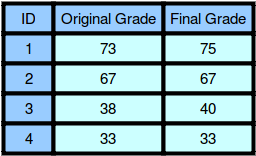HackerLand University has the following grading policy:

• Every student receives a in the inclusive range from to .
• Any less than is a failing grade.

Sam is a professor at the university and likes to round each student’s according to these rules:

• If the difference between the and the next multiple of is less than , round up to the next multiple of .
• If the value of is less than , no rounding occurs as the result will still be a failing grade.

For example, will be rounded to but will not be rounded because the rounding would result in a number that is less than .

Given the initial value of for each of Sam’s students, write code to automate the rounding process. For each , round it according to the rules above and print the result on a new line.

Input Format

The first line contains a single integer denoting (the number of students).
Each line of the subsequent lines contains a single integer, , denoting student ‘s grade.

Constraints

Output Format

For each of the grades, print the rounded grade on a new line.

Sample Input 0

4
73
67
38
33


Sample Output 0

75
67
40
33


Explanation 01. Student received a , and the next multiple of from is . Since , the student’s grade is rounded to .
2. Student received a , and the next multiple of from is . Since , the grade will not be modified and the student’s final grade is .
3. Student received a , and the next multiple of from is . Since , the student’s grade will be rounded to .


<?php

$handle = fopen ("php://stdin","r"); fscanf($handle,"%d",$n); for($a0 = 0; $a0 <$n; $a0++){ fscanf($handle,"%d",$grade);$next = round($grade/5) * 5;$condition = $next -$grade < 3;

if ( $next >$grade && is_float($grade / 5) &&$condition && $grade > 37) { if (!$condition) {
echo $grade."\n"; }else{ echo$next."\n";
}
}else{

1.Maryam malik